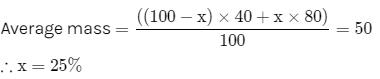Courses

# Test: Mole Concept

## 14 Questions MCQ Test Chemistry for JEE | Test: Mole Concept

Description
This mock test of Test: Mole Concept for JEE helps you for every JEE entrance exam. This contains 14 Multiple Choice Questions for JEE Test: Mole Concept (mcq) to study with solutions a complete question bank. The solved questions answers in this Test: Mole Concept quiz give you a good mix of easy questions and tough questions. JEE students definitely take this Test: Mole Concept exercise for a better result in the exam. You can find other Test: Mole Concept extra questions, long questions & short questions for JEE on EduRev as well by searching above.
QUESTION: 1

### Vapour density of a gas is 22. Its molecular mass will be:​

Solution:

Molecular mass = 2 x vapour density
= 2 x 22
= 44
Therefore, molecular mass of a gas will be 44.

QUESTION: 2

### What is the mass of 0.20 mole of C2H5OH (ethanol)?

Solution:

Molecular Mass of Ethanol
= 2(12) + 5 (1) + 16 + 1
= 24 + 5 + 17
= 46
Now,
Moles (n) = Given Mass (m)/Molecular Mass (M)
Thus, to = nM
= (0.2) (46)
= 9.2

QUESTION: 3

### Consider the reaction between hydrogen and oxygen gases to form water. Which of the following is/are not conserved in the reaction? 2H2(g) + O2(g) → 2H2O(l)​

Solution:

In the equation both side of reaction moles of atoms is conserved ie, both reactant and product contain 4H and 2O but there is 3 (2+1) moles of reactant molecules turns into 2 moles of product molecules.

QUESTION: 4

Mass of sucrose C12H22O11 produced by mixing 84 gm of carbon, 12 gm of hydrogen and 56 lit. O2 at 1 atm & 273 K according to given reaction, is C(s) + H2(g) + O2(g) → C12H22O11 (s)

Solution:

C = 84/12 = 7 mole

H2 = 12 g = 6 mole

O2 = 56/22.4 = 5/2 mole

12C + 11H2 + 11/2 O2 → C12H22O11

L.R. = O2

11/2 mole O2 produce 1 mole sucrose 5/2 mole O2 will for 5/11 mole sucrose mass of sucrose = 5/11 × (mol. mass)

= 5/11 × 342

= 155.45 g

QUESTION: 5

Suppose the elements X and Y combine to form two compounds XY2 and X3Y2. When 0.1 mole of XY2 weighs 10 g and 0.05 mole of X3Y2 weighs 9 g, the atomic weights of X and Y are

Solution:

Let atomic weight of x = Mx

atomic weight of y = My

we know,

mole = weight /atomic weight

a/c to question,

mole of xy2 = 0.1

so,

0.1 = 10g/( Mx +2My)

Mx + 2My = 100g -------(1)

for x3y2 ; mole of x3y2 = 0.05

0.05 = 9/( 3Mx + 2My )

3Mx + 2My = 9/0.05 = 9× 20 = 180 g ---(2)

solve eqns (1) and (2)

2Mx = 80

Mx = 40g/mol

and My = 30g/mole

QUESTION: 6

Number of atoms present in 52 g of helium is:
(NA is Avogadro’s constant Gram atomic mass of He is 4 g)​

Solution:

Gram atomic mass of helium is 4 g so number of atoms present in 52 g of helium
52/4 × NA = 13 NA

QUESTION: 7

The vapour density of a mixture of gas A (Molecular mass = 40) and gas B (Molecular mass = 80) is 25.Then mole % of gas B in the mixture would be

Solution:

As vapour density of mixture is 25,

Molar mass of mixture is 2×25=50.

Now, assume mole % of B in mixture is x.QUESTION: 8

Atomic mass of Cl is 35.5 g. Calculate the mass of 4.50 moles of chlorine gas, Cl2.​

Solution:

Mass of 1 mole of Clis 71 g. Hence mass of 4.50 moles is 71 X 4.50 = 319.5 g

QUESTION: 9

Atomic mass of bromine is 80 g. The mass of four moles of molecular bromine (Br2) is:​

Solution:

Mass of one mole of Br2  is 160g. Hence mass of four moles of molecular bromine is 4 X 160 = 640 g.

QUESTION: 10

Number of nitrogen atoms present in 1.4 g of N2

Solution:

Given weight of N₂ gas = 1.4 g

Molar mass of N₂ gas = 28 g

So, mole = given mass/ molar mass

⇒ mole = 1.4/28 = 1/20 mole

Now, number of molecules = mole × avogadro number

⇒ number of molecules = 1/20 × 6.022 × 10²³

⇒ number of molecules = 3.011 × 10²²

Now, we are asked for number of atoms. In N₂, there are 2 atoms, so to obtain number of atoms we will multiply with 2 in number of molecules.

⇒ number of atoms = 2 × 3.011 × 10²²

⇒ number of atoms = 6.022 × 10²²

QUESTION: 11

Volume of 17g of NH3 at N.T.P. will be​

Solution:

Molar mass of NHis 17g. So the volume of 17g of NH3 i.e 1 mole NH3 at N.T.P. will be 22.4L.

QUESTION: 12

Which of the following has maximum number of moles?​

Solution:

1g hydrogen has the maximum number of moles because atomic mass of hydrogen is smallest among all.

QUESTION: 13

Molar mass of F2 is 38 g. How many atoms are present in 0.147 mole of F2?​

Solution:

One molecule of F2  contains 2 atoms of fluorine.

1 mol of fluorine contains 2 X 6.023 X 1023 atoms

So, 0.147 moles will contain 0.147 X 2 X 6.023 X 1023 = 1.76 X 1023 atoms of flourine.

QUESTION: 14

Mass of 6.022 x 1022 molecules of CO2 is about:​

Solution:

1 mole of a substance is equal to its molecular mass expressed in terms of grams.
Gram molecular mass of CO2 = 12 + (16 Χ 2) = 12 + 32 = 44 g
Mass of 6.023 X1022 molecules of CO2 is 44g/10 = 4.4g.